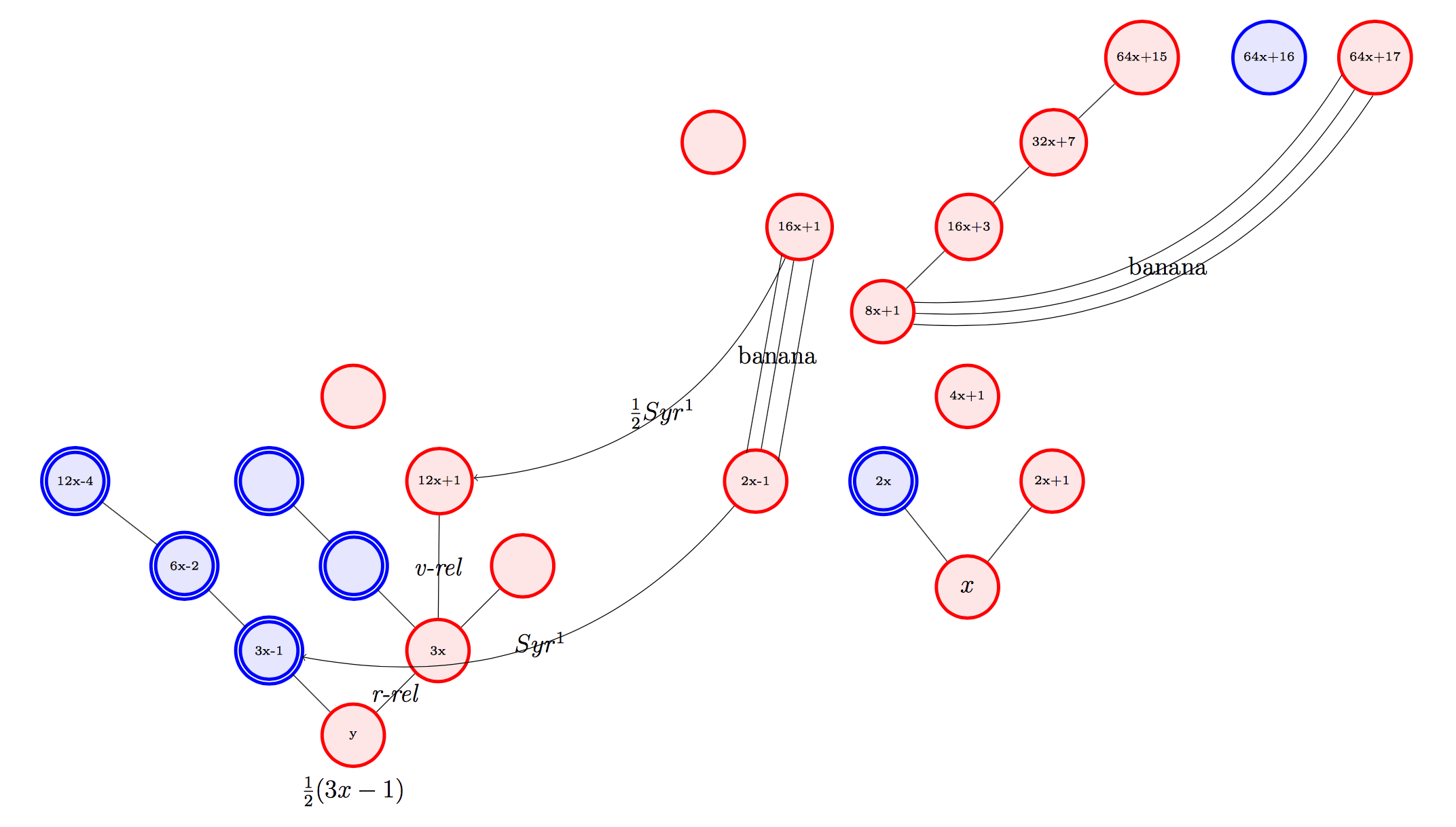# Prépublication : On the Syracuse conjecture over the binary tree

Idriss J. Aberkane  August 15, 2017

Abstract

We investigate recurrent behaviors of the 1 (3x + 1) action (“Syr”) over the binary tree. The original composition of otherwise simple algebraic behaviors (eg: if a = 4b + 1, then Syr(a) = 4(Syr(b))) allows us to identify the existence of a relation merging orbits over alternate pairs of any odd branch of the tree. We prove that it is possible to compress any Syracuse orbits to critical positions in the tree and give new hints as to how to predict those positions. This allows us to establish a novel research program for the resolution of the Collatz conjecture, of which we also introduce original, and simpler conjectures. This article proves that for any even number e, the orbits of V ert(e) := 4e + 1 and S(V ert(e)) := 2(4e + 1) + 1 merge, but also that for any k that is even, Sk(V ert(e)) and Sk+1(V ert(e)) can be proven to merge. For any odd number o, S(V ert(o)) and S2(V ert(o)) also merge, and so do any Sk(V ert(o)) and Sk+1(V ert(o)) for any odd number k. Another significant result is that for any odd number o, either 8o+1 and 16o+1 merge or 8o+1 merges with 16(Vert(o))+1 and 16o+1 merges with 2o − 1. The main result of this paper is that for any odd number o, proving that the orbits of 4o + 1 and 2(4o + 1) + 1 merge will also prove the Collatz conjecture. That such orbits could be systematically proven to merge we call the Golden Gate Conjecture and outline some aspects of a research program attacking this conjecture.

Aberkane_Syracuse_2017

Share: